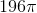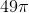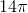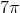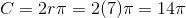ISEE Upper Level Math : Circles

Example Questions

Find the area of a circle with a radius of 6in.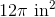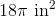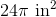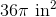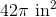Explanation:

To find the area of a circle, we will use the following formula: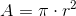Now, we know the radius of the circle is 6in.

Knowing this, we can substitute into the formula.  We get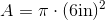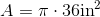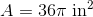Example Question #11 : How To Find The Area Of A Circle

Find the area of a circle with a diameter of 10in.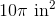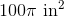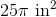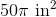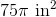Explanation:

To find the area of a circle, we will use the following formula:where is the radius of the circle.

Now, we know the diameter of the circle is 10in.  We also know the diameter is two times the radius.  Therefore, the radius is 5in.

Knowing this, we can substitute into the formula.  We get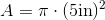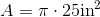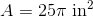Find the area of a circle with a diameter of 14cm.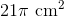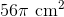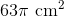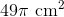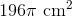Explanation:

To find the area of a circle, we will use the following formula:where r is the radius of the circle.

Now, we know the diameter of the circle is 14cm.  We also know the diameter is two times the radius.  Therefore, the radius is 7cm.

Knowing this, we can substitute into the formula.  We get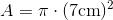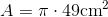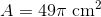You have a tomato plant growing in a pot. If the base of the pot has a radius of 6 inches, find the area of the footprint of the pot.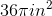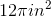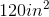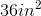Explanation:

You have a tomato plant growing in a pot. If the base of the pot has a radius of 6 inches, find the area of the footprint of the pot.

To solve this problem, first recall the formula for area of a circle.Now, we know our r=6 inches, so we just need to plug in and simplify.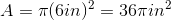Let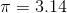.

Find the area of a circle with a diameter of 14cm.  If necessary, round to the nearest tenths.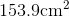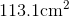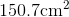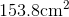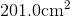Explanation:

To find the area of a circle, we will use the following formula:where r is the radius of the circle.

Now, we know the diameter of the circle is 14cm.  We also know that the diameter is two times the radius.  Therefore, the radius is 7cm.

We also know.

Knowing all of this, we can substitute into the formula.  We get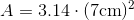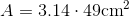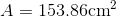We were asked to round to the nearest tenth.  So, we get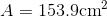Find the area of a circle with a radius of 16in.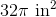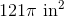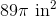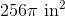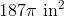Explanation:

To find the area of a circle, we will use the following formula:where r is the radius of the circle.

Now, we know the radius is 16in.  Knowing this, we can substitute into the formula.  We get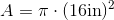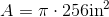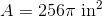You are conducting fieldwork, when you find a tree whose radius at chest height is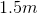. What is the area of a cross section of the tree at chest height?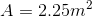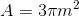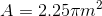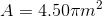Explanation:

You are conducting fieldwork, when you find a tree whose radius at chest height is. What is the area of a cross section of the tree at chest height?

Begin with the formula for area of a circleNow, we know r, so all we have to do is plug it in and solve.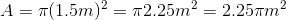Find the area of a circle with a diameter of 16in.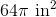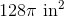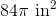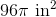Explanation:

To find the area of a circle, we will use the following formula:where r is the radius of the circle.

Now, we know the diameter is 16in. We also know the diameter is two times the radius. Therefore, the radius is 8in. So, we get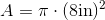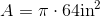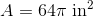A circle has a diameter of 18cm. Find the area.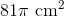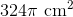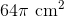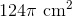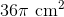Explanation:

To find the area of a circle, we will use the following formula:where r is the radius of the circle.

Now, we know the diameter of the circle is 18cm.  We also know the diameter is two times the radius.  Therefore, the radius is 9cm.

Knowing this, we can substitute into the formula.  We get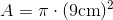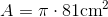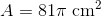Example Question #1 : Circumference Of A Circle

What is the circumference of a circle with a radius equal to?